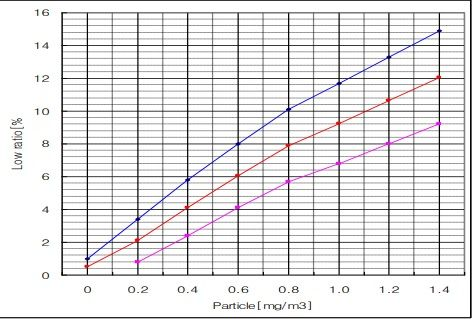# Possible mistake in the code of the exemple DustSensorDSM

• The code shows that the concentration can be calculated by the expression:
long concentration = 1.1pow(ratio,3)-3.8pow(ratio,2)+520ratio+0.62; // using spec sheet curve
But the sheet curve, as we can see in the graph, we have the concentration in the x axis, not in y as in the code:
ratio= 1.1
pow(ratio,3)-3.8pow(ratio,2)+520ratio+0.62
I didn't understand why, in every code i see of this sensor, is the same. Can someone explain?
If it is a dumb question, sorry, but help me please.
The graph of data sheet.
@epierre

• Corrections of the post:
ratio= 1.1pow(concentrarion,3)-3.8pow(conccentration,2)+520*concentration+0.62
the graph3

9

18

6

1

5